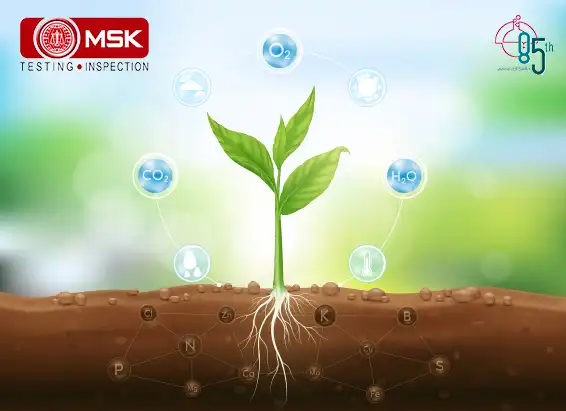Mitra S.K. Private Limited### Determination of Ozone Generation Efficiency of UV Lamp by Chemical Method

Introduction:

The Ultraviolet spectrum has four wavelengths, these are:

• UV-A (400 to 315 nm);
• UV-B (315 to 280 nm);
• UV-C (280 to 200 nm);very high energy and destructive and
• Vacuum UV (200 to 100 nm).

Only this last wavelength, Vacuum UV, is capable of producing ozone.Principle:
This method can be used to determine the efficiency of the UV lamp for generation of Ozone. Generated ozone in a close system by UV lamp is passed through neutral potassium iodide solution at a fixed flow rate. The reaction between ozone and potassium iodide liberates iodine which again reacts with excess potassium iodide to form potassium tri iodide. The formation of triiodide depends on the concentration of ozone produced by the lamp.The produced potassium triiodide is determined by UV-visible spectrophotometer at 352 nm.

1. MATERIALS AND METHODS:

1.1 MATERIALS:

• Ozone generating UV lamp
• PVC pipe
• Suction pump
• Flow meter
• Stop watch
• Midget Impinger
• Impinger box with cooling arrangement
• Silicon tube
• UV -VISIBLE spectrophotometer
• QUARTZ cuvette (10mm)

Absorbing solution (1% KI in 0.1 M Phosphate buffer)  :

13.6 g of potassium dihydrogen phosphate (KH2PO4), 14.2 g of disodium hydrogen phosphate (Na2HPO4) and 10.0 g of potassium iodide are dissolved in sequence to 400 ml distilled water and diluted 1000 ml with distilled water. This solution is to be kept at room temperature for at least 1 day before use. The pH of the solution is adjusted to 6.8 + 0.2 with NaOH or KH2PO4.This solution can be stored for several months in a glass stoppered brown bottle at room temperature without deterioration. It should not be exposed to direct sunlight.

Potassium dichromate (0.05 N):

0.6129 g of Potassium dichromate is dissolved in 100 ml of distilled water and volume made upto 250 ml.

Sodium Thiosulphate (0.05 N) :

3.1025 g of Sodium Thiosulphate is dissolved in 250 ml distilled water.

Starch:

0.2 g Starch and pinch of Mercuric iodide are dissolved in 100 ml boiling distilled water.

Iodine stock solution I2 (0.05 N) :

16 g of potassium iodide and 3.173 g of resublimed iodine are dissolved in 200 ml of distilled water and  diluted 500 ml with distilled water. This solution is to be kept at room temperature for at least 1 day before use.

Standardization of I2 stock Solution:

Sodium thiosulphate (0.05N) is standardised by using standard Potassium dichromate (0.05N). This solution is used for standardisation of iodine solution. Briefly, 10ml of the 0.05 (N) Iodine solution is taken into a 250 ml Iodine flask and titrated against 0.05 (N) Sodium thiosulphate solution using starch indicator.

Normality of Iodine Y(N) = (T.V of Na2S2O3 x strength of Na2S2O3)/ Vm

Vm= Volume of Iodine solution taken in flask (10 ml)

Working standard solution of iodine (0.0025N):

5.0 ml of iodine solution (Y) was diluted to 100 ml with absorbing solution.

Strength of working standard solution (Z) =  (Y X 5.0)/100

This solution is to be prepared on the day of use.

SAMPLING:

UV LAMP was inserted into the PVC pipe. One side must be kept open to get entry of air (ozone free).On the other end a nozzle fitted with silicon tube was fitted which is connected with impinger (Glass) containing absorbing solution. The outlet part of the impinger was connected to the inlet part of the second impinger. The outlet part of the second impinger was connected to the suction pump having arrangement to control flow rate.

For low concentration (expected)

10 ml of absorbing solution was placed in a standard impinger and sample for 60 min at the flow rate of 2 L/min.

For high concentration (expected)

30 ml of absorbing solution was placed in a standard impinger and sample for one hour at the flow rate of 0.5 to 1 L/min.

Exposure of the absorbing reagent to direct sunlight should be strictly restricted.

After sampling, the volume of sample was measured and transferred to a sample storage amber bottle

A) Analysis

If, appreciable evaporation of the absorbing solution occurs during sampling, distilled water was added to bring the liquid volume to 10 ml. The solution was transferred in to the 10mm cuvette & the absorbance was recorded at 352 nm against a reference cuvette containing distilled water.

The absorbance of the unexposed reagent was also recorded and subtracted from the absorbance of the sample.

B) PREPARATION OF STANDARD CURVE

From the working Iodine solution (0.0025N), preparation of a standard solutions were done as per below table and the absorbance were recorded at 352 nm:

 Content O3 (µl/10 ml) absorbance 1.2 0.111 2.4 0.233 4.8 0.395 7.3 0.649 9.8 0.869 12.2 1.138

1.224 *100000 = conversion factor for Normality(N) to µl for 10 ml sample

CALCULATION:

Effective Volume (V2)  of pipe(cc)  = ᴨ(d/2)2l  Where  d= diameter of the tube  in cm(3.81cm), l = effective length of the pipe in cm (77cm)= 877cc

Volume(V1) of UV lamp in the pipe(cc)  = ᴨ(d1/2)2l1  Where  d1= diameter of the UV lamp  in cm(1.5cm), l1 =  length of the UV lamp in cm (77cm)=136 cc

Air Space between the tubes:(V) = V2-V1 = (877 - 136)=741 cc

Volume of Air collected(Va) = flow rate(LPM) * time(min)*1000 = 0.5LPM *1*1000= 500cc

Content of Ozone (C ) in μl= (As – Ab)/ Slope   =( 0.903- 0.388)/0.09185= 280 µl(50 times dilution)

Rate of generation of Ozone(µg/h) =  C*1.962*V* 60/Va*t=280 *1.962*741*60/500*1 =47803µg/hr

Rate of generation of Ozone (mg/h) =  Rate of generation of Ozone (µg/h) /1000    = 47803 µg/1000   =  47.8 mg/h

Where,

As = Absorbance of sample

Ab = Absorbance of reagent blank

Slope =absorbance/μl unit from standard curve=0.0

1.962 = Conversion factor, μl to μgConclusion:

By using this experiment, we can estimate the efficiency for the generation of ozone by UV LAMP.

Contributed by: Bodhisatta Maity

## LATEST blog### Analysis of Red Mud

Posted on December 09 2022 By Mitra S.K ADMIN